# Sieve of Eratosthenes

Given a number n, print all primes smaller than or equal to n. It is also given that n is a small number.

Example:

`Input : n =10Output : 2 3 5 7 Input : n = 20 Output: 2 3 5 7 11 13 17 19`

The sieve of Eratosthenes is one of the most efficient ways to find all primes smaller than n when n is smaller than 10 million or so

Following is the algorithm to find all the prime numbers less than or equal to a given integer n by the Eratosthenes method:

1. Create a list of consecutive integers from 2 to n: (2, 3, 4, ...., n).
2. Initially, let p equal 2, the first prime number.
3. Starting from p2, count up in increments of p and mark each of these numbers greater than or equal to p2 itself in the list. These numbers will be p(p+1)p(p+2)p(p+3), etc..
4. Find the first number greater than p in the list that is not marked. If there was no such number, stop. Otherwise, let p now equal this number (which is the next prime), and repeat from step 3.

When the algorithm terminates, all the numbers in the list that are not marked are prime.

Explanation with Example:
Let us take an example when n = 50. So we need to print all prime numbers smaller than or equal to 50.

We create a list of all numbers from 2 to 50.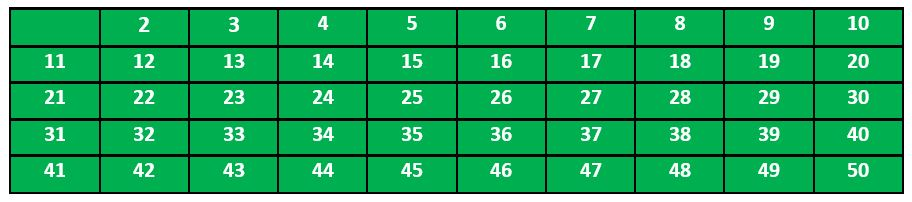According to the algorithm we will mark all the numbers which are divisible by 2 and are greater than or equal to the square of it.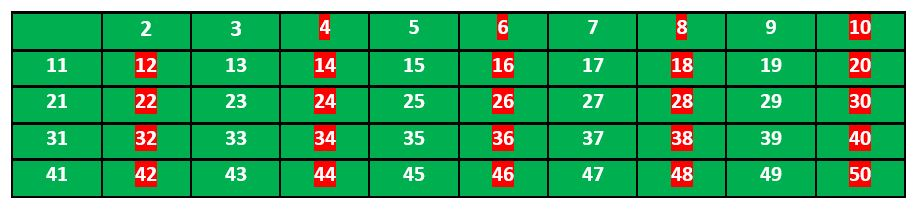Now we move to our next unmarked number 3 and mark all the numbers which are multiples of 3 and are greater than or equal to the square of it.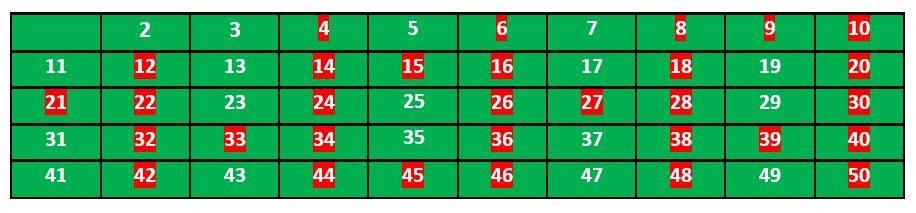We move to our next unmarked number 5 and mark all multiples of 5 and are greater than or equal to the square of it.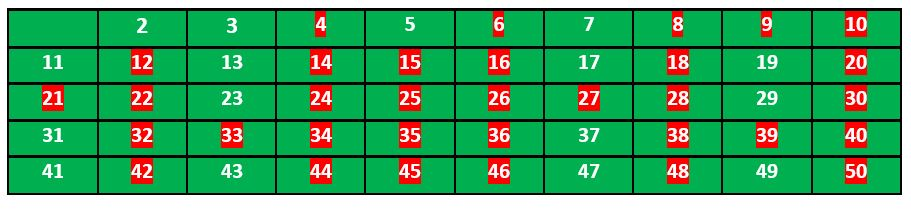We continue this process and our final table will look like below: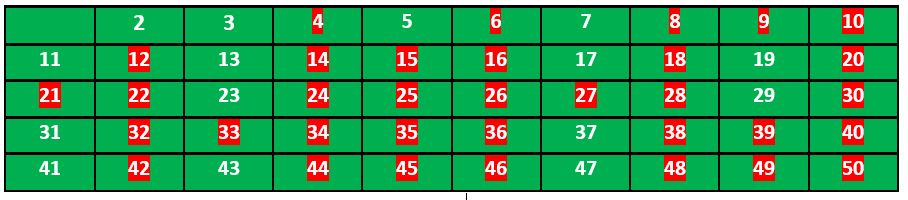So the prime numbers are the unmarked ones: 2, 3, 5, 7, 11, 13, 17, 19, 23, 29, 31, 37, 41, 43, 47.

Implementation:

Following is the implementation of the above algorithm. In the following implementation, a boolean array arr[] of size n is used to mark multiples of prime numbers.

// C++ program to print all primes smaller than or equal to
// n using Sieve of Eratosthenes
#include <bits/stdc++.h>
using namespace std;
void SieveOfEratosthenes(int n)
{
// Create a boolean array "prime[0..n]" and initialize
// all entries it as true. A value in prime[i] will
// finally be false if i is Not a prime, else true.
bool prime[n+1];
memset(prime, true, sizeof(prime));
for (int p=2; p*p<=n; p++)
{
// If prime[p] is not changed, then it is a prime
if (prime[p] == true)
{
// Update all multiples of p greater than or
// equal to the square of it
// numbers which are multiple of p and are
// less than p^2 are already been marked.
for (int i=p*p; i<=n; i += p)
prime[i] = false;
}
}
// Print all prime numbers
for (int p=2; p<=n; p++)
if (prime[p])
cout << p << " ";
}
// Driver Program to test above function
int main()
{
int n = 30;
cout << "Following are the prime numbers smaller "
<< " than or equal to " << n << endl;
SieveOfEratosthenes(n);
return 0;
}

Output:
`Following are the prime numbers smaller than or equal to 302 3 5 7 11 13 17 19 23 29`

Time complexity : O(n*log(log(n)))#### More Articles of M Mounika:

Name Views Likes
C++ Segmented Sieve (Print Primes In a Range) 162 0
C++ Sieve Of Erastosthenes 136 0
C++ Gold Mine Problem 295 0
C++ Merge K Sorted Arrays 117 0
C++ K Centers Problem 240 0
C++ Find Nth Catalan Number 311 0
C++ Inplace Rotate square matrix by 90 degrees 286 0
C++ Find Non Repeating Elements in Array 87 0
C++ Merge Two Binary Trees 121 0
C++ Sum of Numbers From Root To Leaf Paths 89 0
C++ Meta Strings 91 0
C++ Flood Fill Algorithm 402 0
C++ smallest substring with maximum distinct characters 200 0
C++ Smallest window with all characters in string 94 0
C++ Minimum Removal of Characters from string to make its permutation as palindrome 87 0
C++ Minimum characters added at front of string in palindrome conversion 69 0
C++ Number of Bracket Reversals needed to make expression Balanced 72 0
C++ String to Palindrome with Append Function 83 0
C++ WildCard pattern matching 76 0
C++ Anagram substring Search 72 0
C++ Manachars Algorithm 74 0
C++ Search String in Grid 83 0
C++ String Matching(Z Algorithm) 67 0
C++ String Matching(Naive Algorithm) 114 0
C++ String Matching(KMP Algorithm) 141 0
C++ Remove Duplicates From String 111 0
C++ Basics of String Manipulation 85 1
C++ Disjoint Data Structure Cycle Detection 87 0
C++ Problem On Disjoint Data Structures 95 0
C++ Disjoint Data Structures Part3 79 0
Disjoint Data Structures Part2 90 0
Disjoint Data Structures 93 1
C++ Segment Trees 321 2
C++ Trie Cost of Data 291 1
C++ Trie Datastructure 279 1
C++ Greedy Approach Minimum number of coins 526 0
C++ Greedy Approach Maximum height Pyramid 329 1
C++ Greedy Approach String lexicographically largest subsequence 247 0
C++ Greedy Approach Lexicographically largest subsequence 364 0
C++ Greedy Approach Prims MST 398 1
C++ Greedy Approach Krushkals MST 458 1
C++ Greedy Approach N-array maximum sum 334 1
C++ Greedy Approach Policemen Catch Thieves 563 1
C++ Greedy Approach Maximum product Subset 546 1
C++ Greedy Approach Minimum Product Subset 349 1
C++ Greedy Approach Fractional Knapsack 737 1
C++ Greedy Approach-Activity Selection Problem 745 1
C++ Greedy Approach-Egyptian Fractions 640 0
C++ Greedy Approach-Huffman Codes 1031 1
C++ Introduction to Greedy Approach 955 2